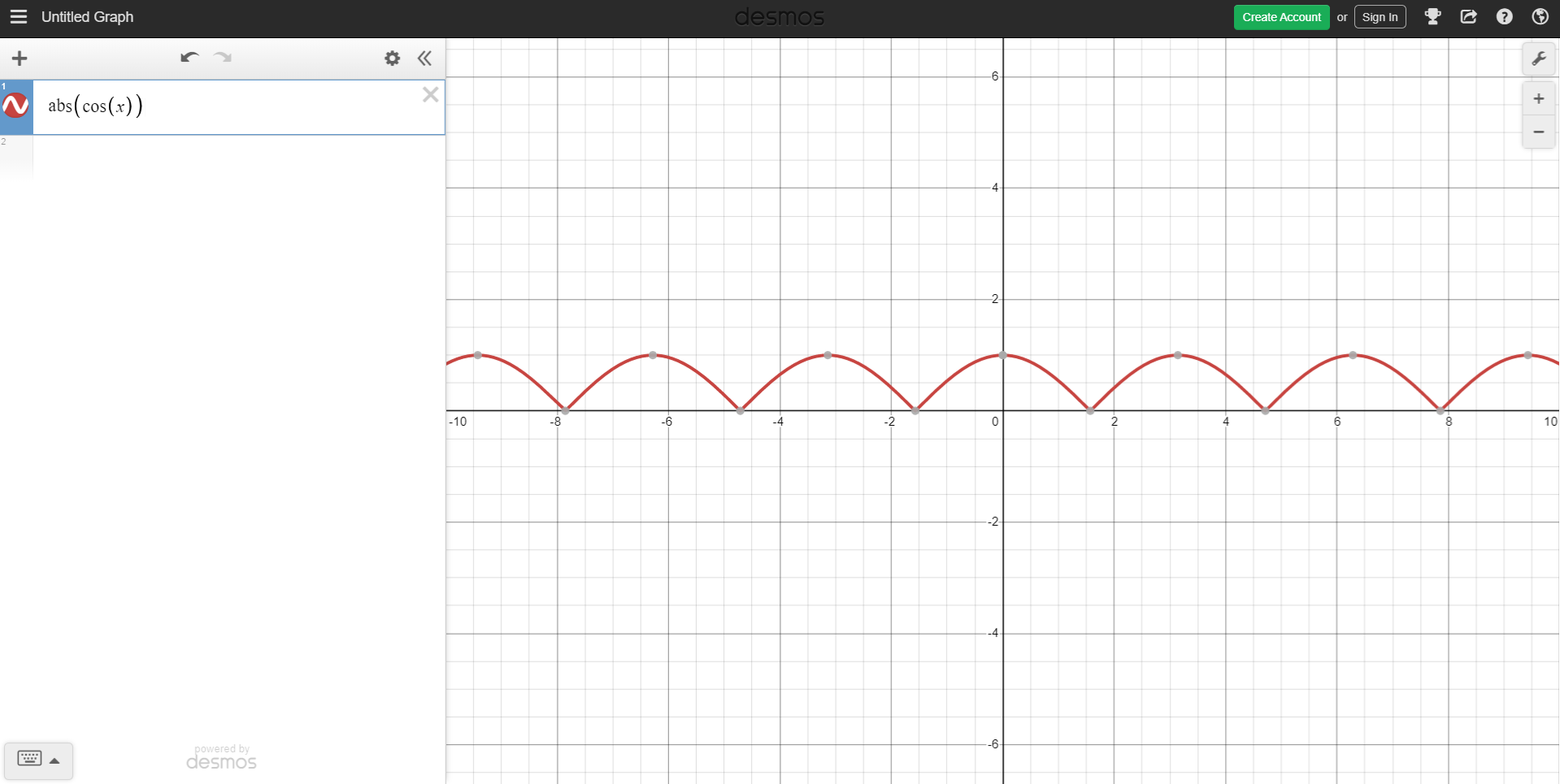# It's kind of like water, but on solid ground.

This post is going to be a little differnt that my last shader post, it'll be more of a direct explaination of the code rather than the process of me figuring out how to make the effect. If you want to see the process, TOO BAD!

Before I post the code I used Unity 2018.3.0f2 and the effect is a screen effect shader. I'm pretty sure you could do the same effect as a grab pass shader but I don't have any good reasons for one implementation over the other. Second, Deleveled is built around having two players that fall in opposite so some of the code is dupicated. If you're looking to use something similar you can probably just rip out anything referencing the bottom player. Third, I'm assuming you know how to write a screen effect shader, if you don't here's where I learned about them. Now, for the full code:

```Shader "Deleveled/Tile/ScreenEffectRipple"
{
Properties
{
_MainTex("Main Texture", 2D) = "white" {}
_TopPosition("Top Position", Vector) = (0, 0, 0, 0)
_TopMaxDistance("Top Max Distance", Vector) = (0, 0, 0, 0)
_TopAmplitude("Top Amplitude", float) = 1
_TopOffsetMultiplier("Top Offset Multiplier", float) = 1
_TopTime("Top Time", float) = 0
_BottomPosition("Bottom Position", Vector) = (0, 0, 0, 0)
_BottomMaxDistance("Bottom Max Distance", Vector) = (0, 0, 0, 0)
_BottomAmplitude("Bottom Amplitude", float) = 1
_BottomOffsetMultiplier("Bottom Offset Multiplier", float) = 1
_BottomTime("Bottom Time", float) = 0
}
{
Pass
{
CGPROGRAM
#pragma vertex vert
#pragma fragment frag

#include "UnityCG.cginc"

struct appdata
{
float4 vertex : POSITION;
float2 uv : TEXCOORD0;
};

struct v2f
{
float4 vertex : SV_POSITION;
float2 uv : TEXCOORD0;
float4 worldPos : TEXCOORD1;
};

sampler2D _MainTex;
float4 _MainTex_ST;
float4x4 _ScreenToWorld;

float4 _TopPosition;
float4 _TopMaxDistance;
float _TopAmplitude;
float _TopOffsetMultiplier;
float _TopTime;

float4 _BottomPosition;
float4 _BottomMaxDistance;
float _BottomAmplitude;
float _BottomOffsetMultiplier;
float _BottomTime;

v2f vert(appdata v)
{
v2f o;
o.vertex = UnityObjectToClipPos(v.vertex);
float4 clipVert = o.vertex;
o.worldPos = mul(_ScreenToWorld, clipVert);
o.worldPos /= o.worldPos.w;
o.uv = TRANSFORM_TEX(v.uv, _MainTex);
return o;
}

float getDistortion(float4 worldPosition, float4 ripplePosition, float4 maxDistance, float amplitude, float time, int signMultiplier)
{
float2 distance = float2(abs(worldPosition.x - ripplePosition.x), -signMultiplier * (worldPosition.y - ripplePosition.y));
fixed waveMask = smoothstep(1, 0, distance.x / maxDistance.x);

//Don't render the wave if it is lower than the position
//Don't render the wave if it is too high above the position
waveMask *= step(distance.y, -signMultiplier * maxDistance.y);
return amplitude * signMultiplier * cos(distance.x + time) * waveMask;
}

fixed4 frag(v2f i) : SV_Target
{
i.uv.y -= getDistortion(i.worldPos, _TopPosition, _TopMaxDistance, _TopAmplitude, _TopTime, -1) * _TopOffsetMultiplier;
i.uv.y -= getDistortion(i.worldPos, _BottomPosition, _BottomMaxDistance, _BottomAmplitude, _BottomTime, 1) * _BottomOffsetMultiplier;
return tex2D(_MainTex, i.uv);
}
ENDCG
}
}
}
```

## Properties

• _MainTex - Current image on the screen.
• _Top/BottomPosition - The Vector2 position the top/bottom player has landed.
• _Top/BottomMaxDistance - The Vector2 range the ripple will distort. For example, if you want the ripple to affect 3 world units on the x-axis and 1 on the y-axis, you'd pass in (3, 1).
• _Top/BottomAmplitude - The ripple is basically a sine wave, so this is how crazy the distortion is on the y axis.
• _Top/BottomOffsetMultiplier - This property is rather silly as it's basically the same as the amplitude but having the property made thinking about how the effect behaves over time easier for me.
• _Top/BottomTime - Animate the wave. This is similar to using _Time in the shader, but it was easier to manually reset the timer a 0 with each new bounce.

## Algorithm

As hinted at above, the algorithm behind the affect is a mirrored sine wave that expands on the x-axis (via the _Top/BottomMaxDistance property) and shrinks on the y-axis (via the _Top/BottomOffsetMultiplier property) over time. To get a better visual of the actual equation, here is the base equation abs(cos()). Those paying attention may notice that it doesn't exactly look like a wave. And you'd be correct! However, I found that using the absolute value of cos gave a better ripple feel than just offsetting a base cos wave.The next step of the process is applying a mask to the wave (generating the mask is done on lines 70). The main reason for this is so the x-axis edges of the ripple blend together between being rippled and not rippled. I should add, there's also lines 73 and 75 that modify the mask on the y-axis. This is done to isolate the effect to a small section of the screen, if this wasn't there, everything above and below the ground would also rippled. The final step is sampling the image. As you can see on lines 81 - 83 we offset the uv position to "stretch" the image based on the wave equation.

## Controlling The Values

If you've used shaders and materials in Unity, you know that you can set values via C# scripts. For the ripple there's three values being controlled: Time, OffsetMultiplier, and MaxDistance. Time and MaxDistance both start at 0 and increase with time while OffsetMultiplier starts at some value and decreases to 0. Once OffsetMultiplier reaches 0, there's no more ripple. There's lots of logic specific to Deleveled in the C# code (for example, the faster the players are traveling when they hit the ground, the bigger the ripple) so I will not be sharing that mess of code :)

This effect took quite a bit of work to figure out and fine tune so hopefully someone else out there will find this helpful!